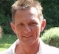## Do floats provide enough resolution to store time? Or do they suffer from "Virtual Digital Jitter" because their time resolution isn't good enough?Started by 3 years ago6 replieslatest reply 3 years ago152 views
Hi, My understanding of jitter is that it happens when the timing of the analog-to-digital converter is off. But what about computer generated sine waves that store time t in a float: A*sin(2*pi*f*t)? A 32 bit float has a significand of just 23 bits. A nanosecond is 1/10^9, which is around 1/2^30. So, it appears that a 32 bit float only has sub-nanosecond resolution for only 1/128th of a second. Am I misunderstanding this? Do computer generated sine waves suffer from a "virtual jitter" if the floating point's significand isn't large enough to provide enough time resolution?
[ - ]You're right, there's a lot of problems if computers deal with time, especially if they have to derive different time-related values with different representations.

But in your example you can approach it from a very easy understanding:
time is relative. This means:
you start from time=0 and then, usually, you increase the time value by equidistant portions (sample/time interval T).

This means that you take k=2*pi*f*T, calculate it with your available precision.
Now, every next sine value is calculated as A*sin(k*1), A*sin(k*2), A*sin(k*3), and issued after time intervals of T.

As you see, the error contribution now comes from

1. calculating/representing k
2. multiplication of k*n
3. retrieving the sine of a value
4. multiplication with A

If you simply calculate this as you might do it with a desktop calculator, you'd end up with increasing errors while time advances, because your values of k*n increase.

Intelligent algorithms minimize the error. In your case you might retrieve the next value of k*(n+1) by adding k to the previous value, then subtract a value of 2*pi of the result of k*n, if it's big enough; like a floating version of modulo: k(n+1)=((k*n)+k)mod(2pi). This would set boundaries to the value and limit the error.

If the sine wave generator would run for a long time, there might be an increasing phase error. So never rely on a sine wave generator or a time measurement unit like a clock to be mathematically exact. That's why you have to set/adjust every clock after a certain amount of time...

[ - ]I thought what matters for time accuracy is the sampling clock jitter and as long as we can count on this clock using a suitable counter width then the error does not build up.The actual value at any moment can be passed to a register of suitable width. So in terms of hardware implementation I don’t see why float comes here in this discussion.
[ - ]In addition to the previous comments, I'd point out that the resolution of the sine function itself is a problem to think about.  No matter what the argument is, the formula used to compute the sine also has "jitter" because the coefficients of the polynomial are finite.  People have spent a lot of time (like the past 300 years or so) figuring out how to make this the best possible, but because the size of a float only has so many bits you only get to be so accurate.  So on top of the input accuracy, you have to think about the function accuracy as well.  If you really want 1/10^9 accuracy, you should ensure double precision (1/2^54 ~ 1/10^16) computation in the function.

[ - ]Everything that's been said, plus:

It is simply your responsibility, as the system designer, to make sure that you use appropriate algorithms, and data paths that are wide enough to get the job done.  The first thing I'd suggest if you're on a desktop is double-wide floats; if you're in an embedded system there are various ways of generating a phase reference that's more efficient than floating point.

And, ultimately, if your computer-generated sine wave is going to be used to generate a *real* sine wave, or to analyze some real signal that's been acquired, your computer-generated jitter is going to be of diminishing importance as it gets down to, and then below, any timing error in the hardware, or timing induced by the universe (i.e., doppler shift, or lifting your super-accurate atomic-fountain clock onto a table without re-calibrating for the change in gravity).

So there are always limits that you can design to -- and if you don't know those limits, then you know your next task!

[ - ]FWIW, I'm a hardware guy, and these days with Global Navigation Satellite Systems (GNSS, the most familiar probably being Global Positioning System, GPS) so common and available, it's relatively inexpensive to buy a GPS-disciplined oscillator (GPSDO).  I bought one from the UK for about $150, along with a distribution amplifier for about$90, put up an antenna in my garage where it can "see" the sky, and now I have atomic clock accuracy, about 10e-12, as a frequency reference for my test equipment.  The GPSDO is about the size of a cigarette pack and the distribution amp is a bit larger, mostly to accommodate the 9 BNC connectors (1 for input and 8 for outputs) and the DC power input.